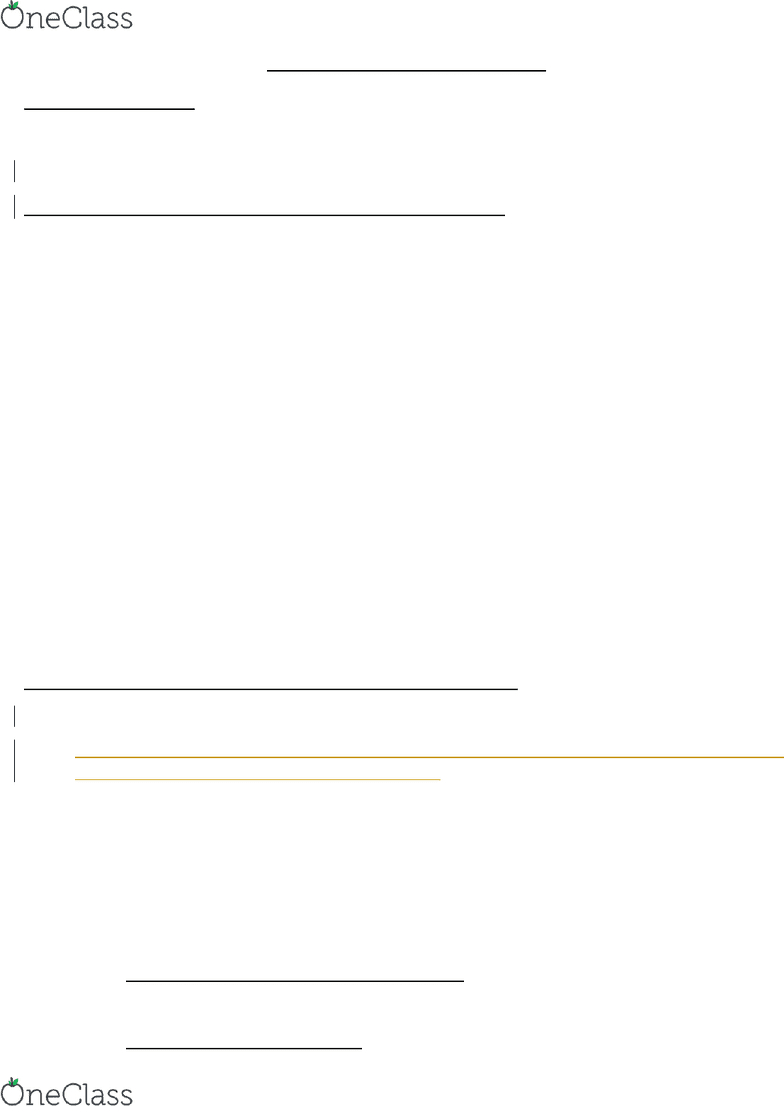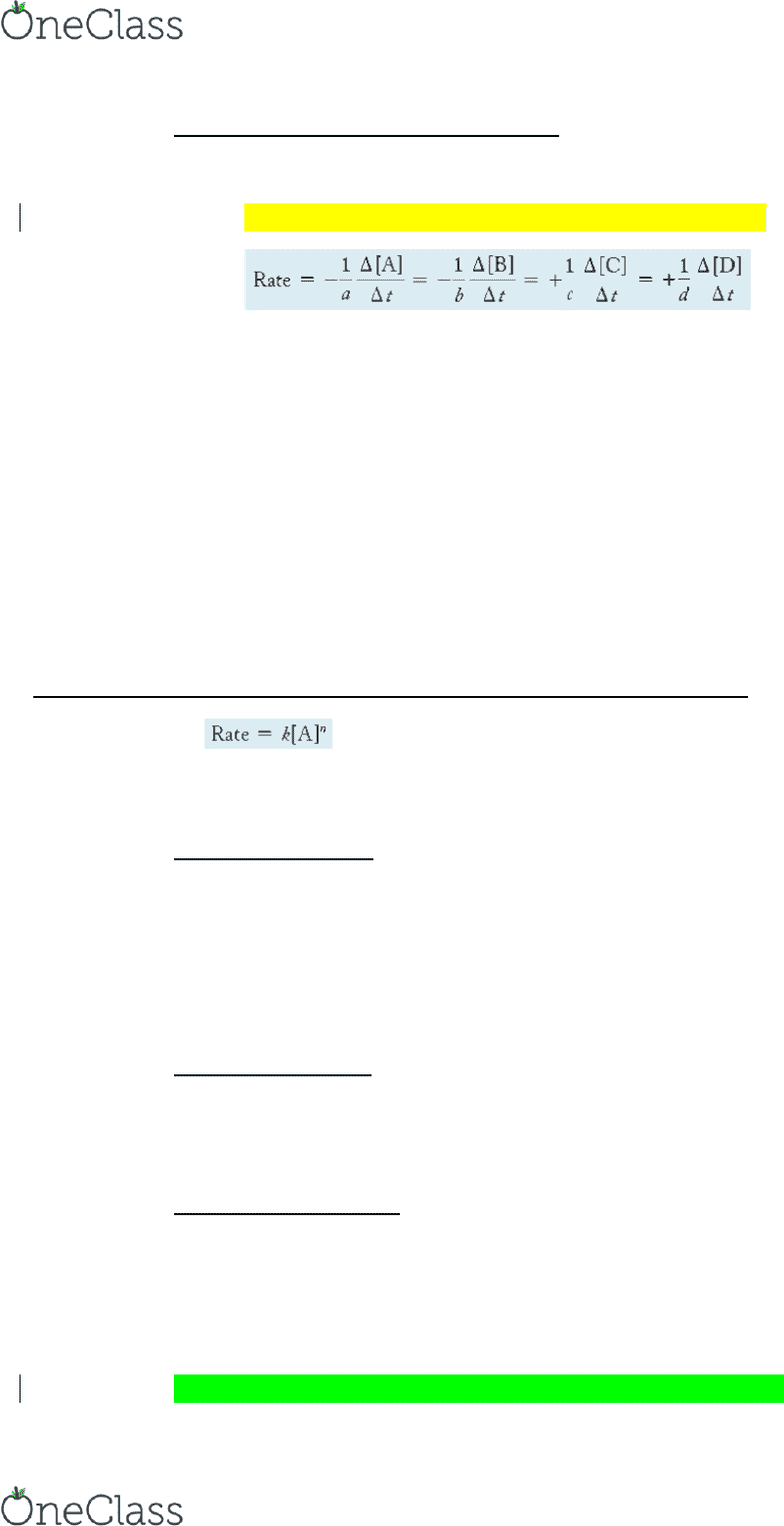Textbook Notes (290,000)
US (110,000)
UC-Irvine (3,000)
CHEM (200)
CHEM 1C (20)
Chapter 15

# CHEM 1C Chapter Notes - Chapter 15: Hydrogenation, Activated Complex, Reaction Intermediate

Department
Chemistry
Course Code
CHEM 1C
Professor
Eric Potma
Chapter
15

This preview shows page 1. to view the full 5 pages of the document.Chapter 15: Chemical Kinetics
15.1 Catching Lizards
Ectotherms= body temperature depends on their surroundings
Reaction rates are very important, and controlling them can be very useful
15.2 Rates of Reaction and the Particulate Nature of Matter
Collision model= the reaction occurs as a result of a collision between A-A particles and B particles
Reaction rate depends on a) concentration, b) temperature, c) structure and orientation
The Concentration of the Reactant Particles
oConcentration = number of particles in a given volume
oGreater the concentration the more collisions between particles, therefore the faster the rate
oLudwig Wilhelmy accurately measured this effect (1850 sucrose experiment)
The Temperature of the Reactant Mixture
oThe initial breaking of the A-A bond requires energy > energy by forming A-B bond
oTherefore, initial or activation energy is required, comes from kinetic energy of collisions
oReaction rate increases with increasing Temperature
The Structure and Orientation of the Colliding Particles
oA and B must form a Bond not B and some other element
oTherefore, Structure and Orientation are key for rate of a reaction
15.3 Defining and Measuring the Rate of a Chemical Reaction
Rate of a chemical reaction is a measure of how fast the reaction occurs
Reaction rate = is the number (mol) of chemical reactions occurring in the system per reaction volume
(L) per time (s). Change in molarity over time (mol/Ls
Defining Reaction Rate
oRate usually defined as change in some quantity per unit of time
oChemical reaction = change in amounts of reactants or products divided by change in time
oChange in the concentration of a reactant is negative
oChange in the concentration of a product is positive
oChange in Reactant and Product Concentrations
Reactant concentration decreases with time, product concentration increases with time
oThe Average Rate of the Reaction
find more resources at oneclass.com
find more resources at oneclass.com

Unlock to view full version

Only page 1 are available for preview. Some parts have been intentionally blurred.Average Rate decreases as the reaction progresses, less concentration as reaction occurs
oThe Instantaneous Rate of the Reaction
Instantaneous rate of the reaction is the rate at any one point in time (derivative)
Rate definition for generic reaction: aA + bB  cC + dD
Predicting the rate at some future time is not possible from just the balanced equation
Measuring Reaction Rates
oPolarimetry = measuring degree of polarization of light passing through a reacting solution
oMost common way to study kinetics of a reaction is through spectroscopy
oMeasure intensity of light absorption, should decrease as reaction proceeds
oAlso monitor changes in pressure, as amount of gas increases, pressure increases
oSome reactions are so slow that samples (aliquots) can be periodically withdrawn and examined
15.4 The Rate Law: The Effect of Concentration on Reaction Rate
Rate Law:
Rate Constant = k constant of proportionality Reaction order = n
oZero-Order Reaction
Rate of the reaction is independent of the concentration of the reactant
The concentration of the reactant decreases linearly with time, slope is constant
Amount of reactant available for reaction is unaffected by changes of overall quantity of
reactant
oFirst-Order Reaction
Rate of the reaction is directly proportional to the concentration of the reactant
Rate slows down as the reaction proceeds, linear relationship
oSecond-Order Reaction
Rate of the reaction is proportional to the square of the concentration of the reactant
Quadratic relationship as the slope of the curve flattens out more quickly
Determining the Order of a Reaction
oThe order of a reaction can be determined only by experiment.
oCommon way to determine is method of initial rates
find more resources at oneclass.com
find more resources at oneclass.com